# Optimize Using the GPS Algorithm

This example shows how to solve an optimization problem using the GPS algorithm, which is the default for the `patternsearch` solver. The example uses the `Optimize` Live Editor task to complete the optimization using a visual approach.

### Objective Function

This example uses the objective function `ps_example`, which is included when you run this example. View the code for the function.

`type ps_example`
```function f = ps_example(x) %PS_EXAMPLE objective function for patternsearch. % Copyright 2003-2021 The MathWorks, Inc. f = zeros(1,size(x,1)); for i = 1:size(x,1) if x(i,1) < -5 f(i) = (x(i,1)+5)^2 + abs(x(i,2)); elseif x(i,1) < -3 f(i) = -2*sin(x(i,1)) + abs(x(i,2)); elseif x(i,1) < 0 f(i) = 0.5*x(i,1) + 2 + abs(x(i,2)); elseif x(i,1) >= 0 f(i) = .3*sqrt(x(i,1)) + 5/2 +abs(x(i,2)); end end ```

Plot the function.

```fsurf(@(x,y)reshape(ps_example([x(:),y(:)]),size(x)),... [-6 2 -4 4],'LineStyle','none','MeshDensity',300) colormap 'jet' view(-26,43) xlabel('x(1)') ylabel('x(2)') title('ps\_example(x)')```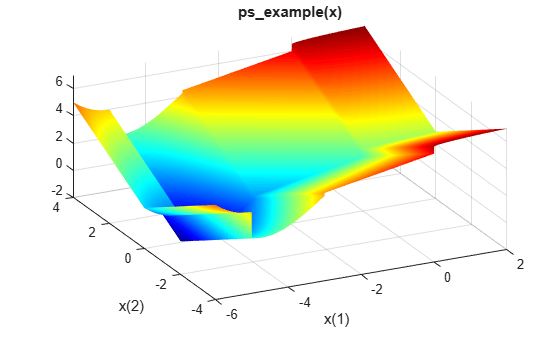### Find the Minimum of the Function

To find the minimum of `ps_example` using the `Optimize` Live Editor task, complete the following steps.

• Create a new live script by clicking the New Live Script button in the File section on the Home tab.• Insert an `Optimize` Live Editor task. Click the Insert tab and then, in the Code section, select Task > Optimize.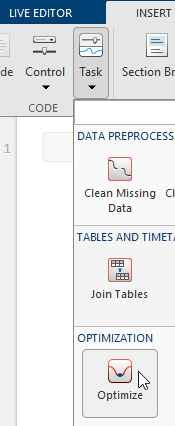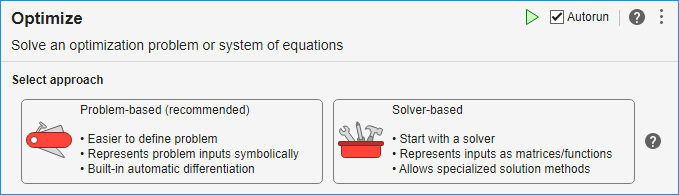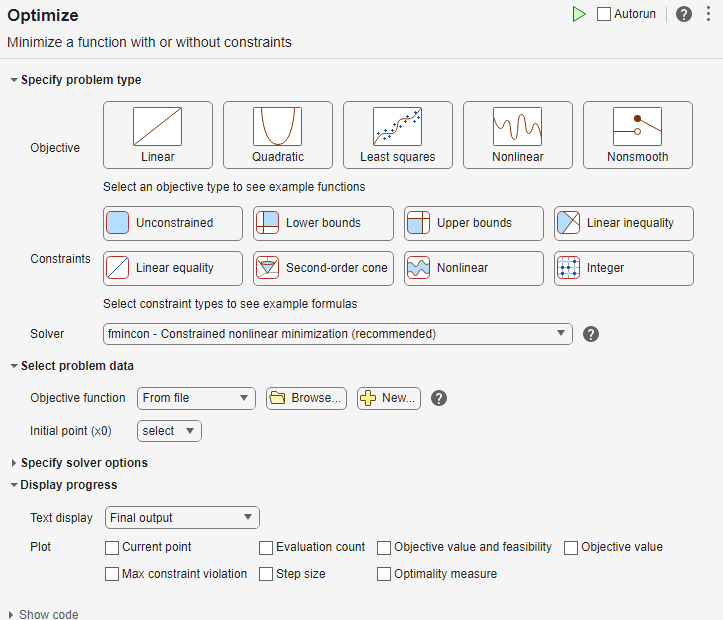• For use in entering problem data, insert a new section by clicking the Section Break button on the Insert tab. New sections appear above and below the task.

• In the new section above the task, enter the following code to define the initial point and objective function.

```x0 = [2.1 1.7]; fun = @ps_example;```
• To place these variables into the workspace, run the section by pressing Ctrl + Enter.

• In the Specify problem type section of the task, click the Objective > Nonsmooth button.

• Ensure that the selected solver is `patternsearch`.

• In the Select problem data section of the task, select Objective function > Function handle and then choose `fun`.

• Select Initial point (x0) > x0.

• In the Display progress section of the task, select the Best value and Mesh size plots.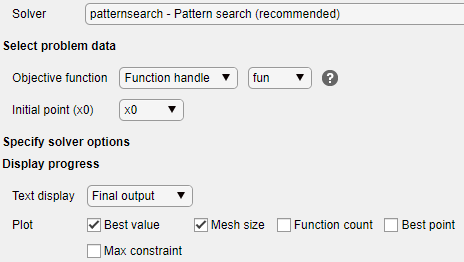• To run the solver, click the options button at the top right of the task window, and select Run Section. The plots appear in a separate figure window and in the task output area.The upper plot shows the objective function value of the best point at each iteration. Typically, the objective function values improve rapidly at the early iterations and then level off as they approach the optimal value.

The lower plot shows the mesh size at each iteration. The mesh size increases after each successful iteration and decreases after each unsuccessful iteration. For details, see How Pattern Search Polling Works.

The optimization stops because the mesh size becomes smaller than the mesh size tolerance value, defined by the `MeshTolerance` option. The minimum function value is approximately –2.

To see the solution and objective function value, look at the top of the task.The `Optimize` task puts the variables `solution` and `objectiveValue` in the workspace. View these values by placing a new section below the task, and include this code.

```disp(solution) disp(objectiveValue) ```

Run the section by pressing Ctrl+Enter.

The Optimize Live Editor task appears next in its final state.

``````
```Optimization terminated: mesh size less than options.MeshTolerance. ```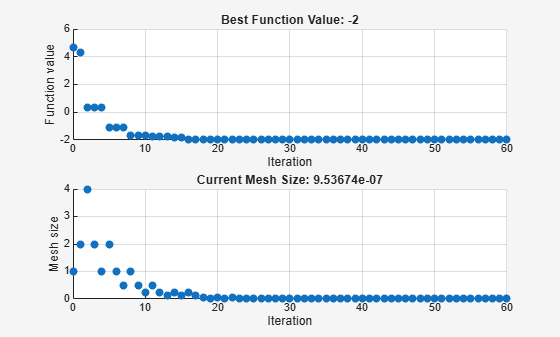`disp(solution)`
``` -4.7124 -0.0000 ```
`disp(objectiveValue)`
``` -2.0000 ```